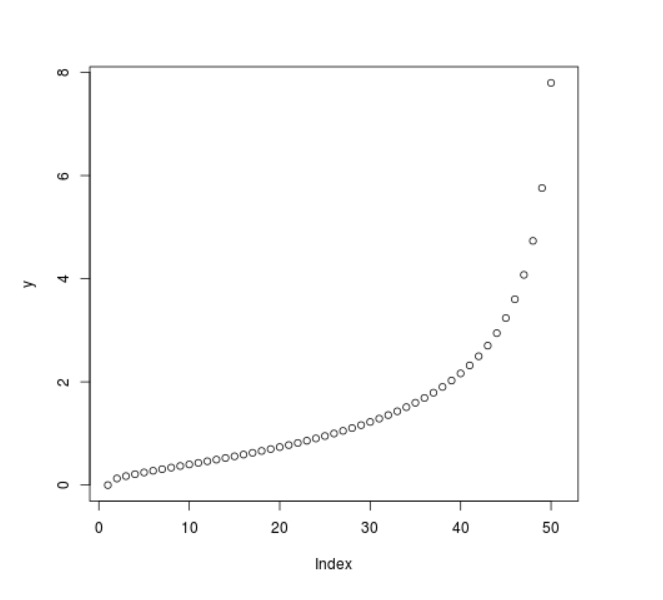Related Articles

# Compute value of Log Normal Quantile Function in R Programming – qlnorm() Function

• Last Updated : 25 Jun, 2020

`qlnorm()` function in R Language is used to compute the value of log normal quantile function. It also creates a plot of the quantile function of log normal distribution.

Syntax: qlnorm(vec)

Parameters:
vec: x-values for normal density

Example 1:

 `# R program to compute value of``# log normal quantile function`` ` `# Creating x-values for density``x <``-` `seq(``0``, ``1``, by ``=` `0.2``)`` ` `# Calling qlnorm() function``y <``-` `qlnorm(x)``y`

Output:

``` 0.0000000 0.4310112 0.7761984 1.2883304 2.3201254       Inf
```

Example 2:

 `# R program to compute value of``# log normal quantile function`` ` `# Creating x-values for density``x <``-` `seq(``0``, ``1``, by ``=` `0.02``)`` ` `# Calling qlnorm() function``y <``-` `qlnorm(x)`` ` `# Plot a graph``plot(y)`

Output:My Personal Notes arrow_drop_up